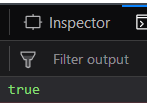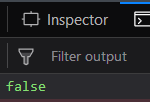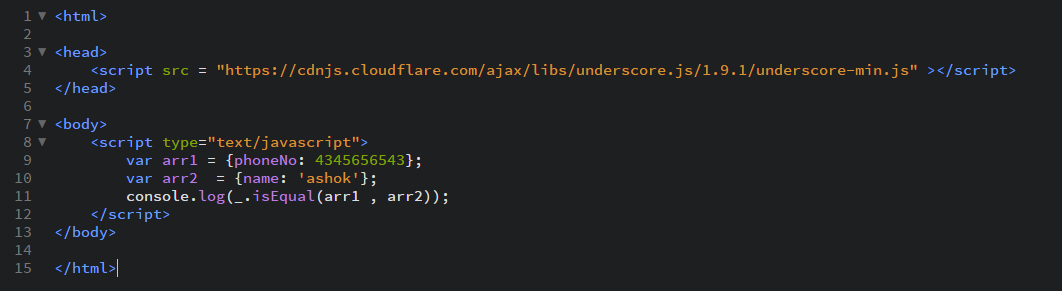GeeksforGeeks App
Open AppBrowser
Continue

# Underscore.js _.isEqual() Function

The _.isEqual() function: is used to find whether the 2 given arrays are the same or not. Two arrays are the same if they have the same number of elements, both the property and the values need to be the same. It may be beneficial in situations where the elements of the array are not known and we want to check whether they are the same or not.

Syntax:

`_.isEqual(object, other)`

Parameters:

It takes two arguments:

• object: The object can be an array.
• other: The other array holds.

Return value: It returns true if the arrays passed are the same otherwise it returns false.

Examples:

Passing 2 simple arrays to the _.isEqual() function:

The _.isEqual() function takes the element from the list of one array and searches it in the other array. If that property is found with the same value in the other array then it just goes on to check the other property otherwise it just returns false. In this, it checks for both the character values and the number values in the property.

## html

 ```<``html``>`` ` `<``head``>``   ``<``script` `src =``"https://cdnjs.cloudflare.com/ajax/libs/underscore.js/1.9.1/underscore-min.js">``   ``````<``body``>``    ``<``script` `type="text/javascript">``        ``var arr1 = {name: 'akash', numbers: [3, 7, 14]};``        ``var arr2  = {name: 'akash', numbers: [3, 7, 14]};``        ``console.log(_.isEqual(arr1, arr2));``    ```````

Output:Passing an array with more properties to the _.isEqual() function:

An array can have as many properties as it has to act as a parameter to this function. Like here both the arrays contain 3 properties each of type character and date. The _.isEqual() function will work in the same way as for the above example. Since both the arrays have the same properties and the same values so, the output will be ‘true’.

## html

 ```<``html``>`` ` `<``head``>``    ``<``script` `src =``    ``"https://cdnjs.cloudflare.com/ajax/libs/underscore.js/1.9.1/underscore-min.js">``    `````` ` `<``body``>``    ``<``script` `type="text/javascript">``        ``var arr1 = {name: 'akash', gender: ['male'], birthDate: [03/22/99]};``        ``var arr2  = {name: 'akash', gender: ['male'], birthDate: [03/22/99]};``        ``console.log(_.isEqual(arr1, arr2));``    `````` ` ``

Output:Passing 2 empty arrays to the _.isEqual() function:

The _.isEqual() function will try to check all the array properties along with their values. Since both the array does not have any property so, there is nothing to match. And hence, both arrays are equal. Therefore, the answer will be true.

## html

 `<``html``>`` ` `<``head``>``    ``<``script` `src =``    ``"https://cdnjs.cloudflare.com/ajax/libs/underscore.js/1.9.1/underscore-min.js">``     `````` ` `<``body``>``    ``<``script` `type="text/javascript">``        ``var arr1 = {};``        ``var arr2  = {};``        ``console.log(_.isEqual(arr1, arr2));``    `````` ` ``

Output:Passing arrays with different properties to the _.isEqual() function:

If we pass arrays containing different properties then this function will work the same way. It will take the property of the first parameter array ( here, ‘name’) and try to find it in the next array. But since the other array does not have this property so the output will be ‘false’.

## html

 `` `<``html``>`` ` `<``head``>``    ``<``script` `src =``    ``"https://cdnjs.cloudflare.com/ajax/libs/underscore.js/1.9.1/underscore-min.js">``    `````` ` `<``body``>``    ``<``script` `type="text/javascript">``        ``var arr1 = {phoneNo: 4345656543};``        ``var arr2  = {name: 'ashok'};``        ``console.log(_.isEqual(arr1, arr2));``    `````` ` ``

Output:```<``script` `type="text/javascript" src =``"https://cdnjs.cloudflare.com/ajax/libs/underscore.js/1.9.1/underscore-min.js">```